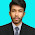WFU「我想做好佢，但大腦成日諗住：聽日先做啦」以下5個方法幫你解決拖延症。

1        你要有一個sense

"You wont get much work done if you only do it when you feel like it."

1        休息的方法

"A typical man should use this time as an aristocrat would: to perform rigorous self improvement."

1. 無論我的狀態如何，我都要完成這件事
2. 我所有的休息時間，我都要用一定的時間在自我提升上。

1        每日5分鐘做計劃

 10:00 - 11:00 食早餐 11:00 - 1:00 做中文PP 1:00 - 3:00 Lunch

To remember:

1        朋友間互相督促

1        增加自己的儀式感

1        每個人的生理時鐘都不同

### 4 留言

1.I wish it is helpful for my remaining two days of econ

2.多謝 :))))))))))))))))))))))))))))))))))))))))))))))))))))))))))))))))))))))))))))))))))))))))))))))))))))))！！

3.好彩有呢篇嘢
又好彩DSE延遲
對DSE開考前追返晒啲嘢有番信心

4.<> Account Age 1 year+
<> 4+ Unique profile Photo
<> School, University, Work, Home city, Current city, should be added to Profile
<> Haven’t any Friends
<> USA male or female profile
<> 100% Real & active Manual Work
<> Blazing fast delivery
<> 12-24 hour delivery time
<> 24 hours customer support
<> Works procedure 100% Right way & Safest Promotion Techniques
<> Affordable Prices
<> 100% money-back guaranteed